# Internal node number

Some of the functions in `ggtree` work with clade and accept a parameter of internal node number. To get the internal node number, user can use `geom_text2` to display it:

``````nwk <- system.file("extdata", "sample.nwk", package="ggtree")
ggtree(tree) + geom_text2(aes(subset=!isTip, label=node), hjust=-.3) + geom_tiplab()``````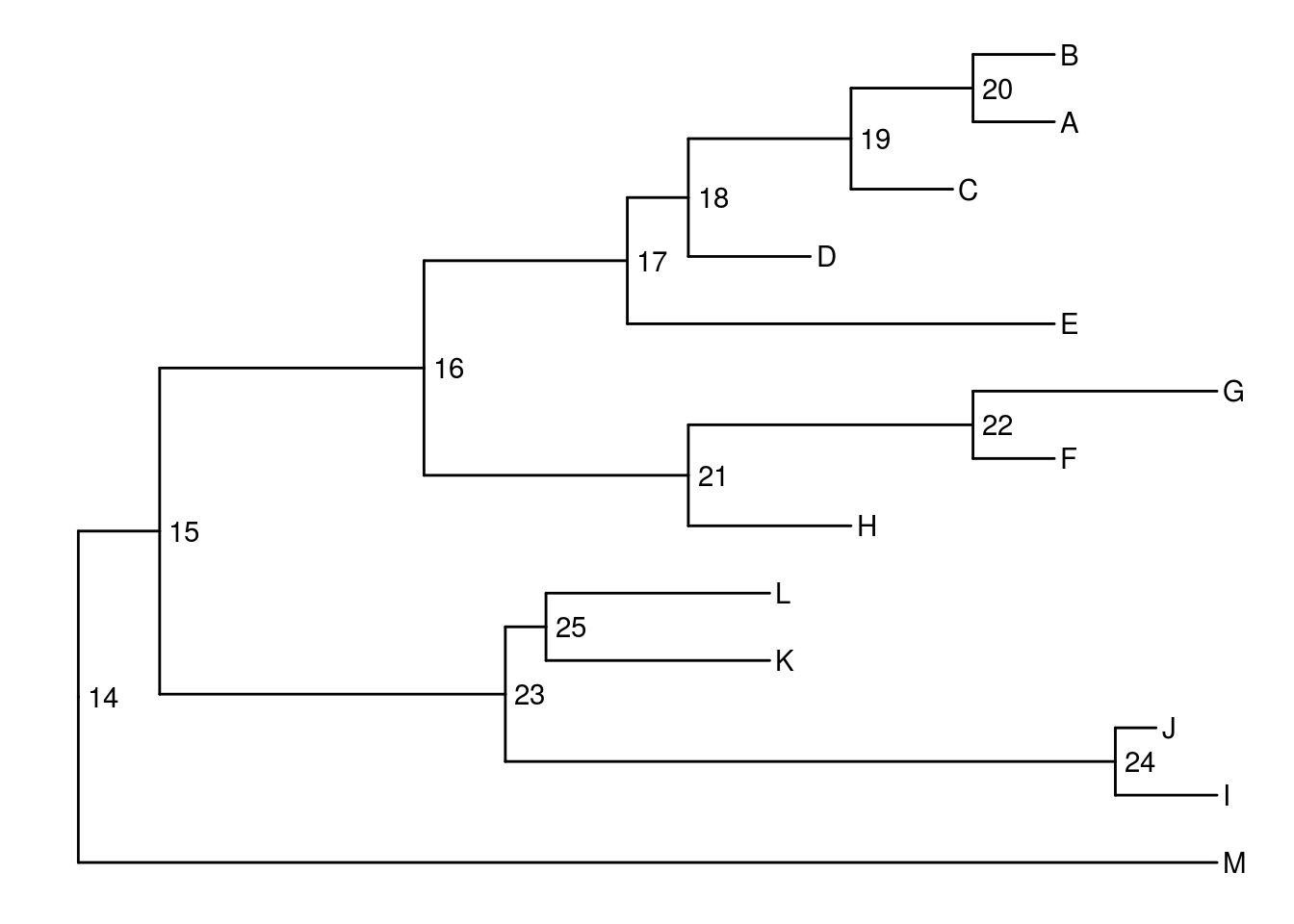Another way to get the internal node number is using `MRCA()` function by providing a vector of taxa names. The function will return node number of input taxa’s most recent commond ancestor (MRCA). It works with tree and graphic object.

``MRCA(tree, tip=c('A', 'E'))``
``##  17``
``MRCA(tree, tip=c('H', 'G'))``
``##  21``
``````p <- ggtree(tree)
MRCA(p, tip=c('A', 'E'))``````
``##  17``

`ggtree` provides a function `viewClade` to visualize a clade of a phylogenetic tree.

``viewClade(p+geom_tiplab(), node=21)``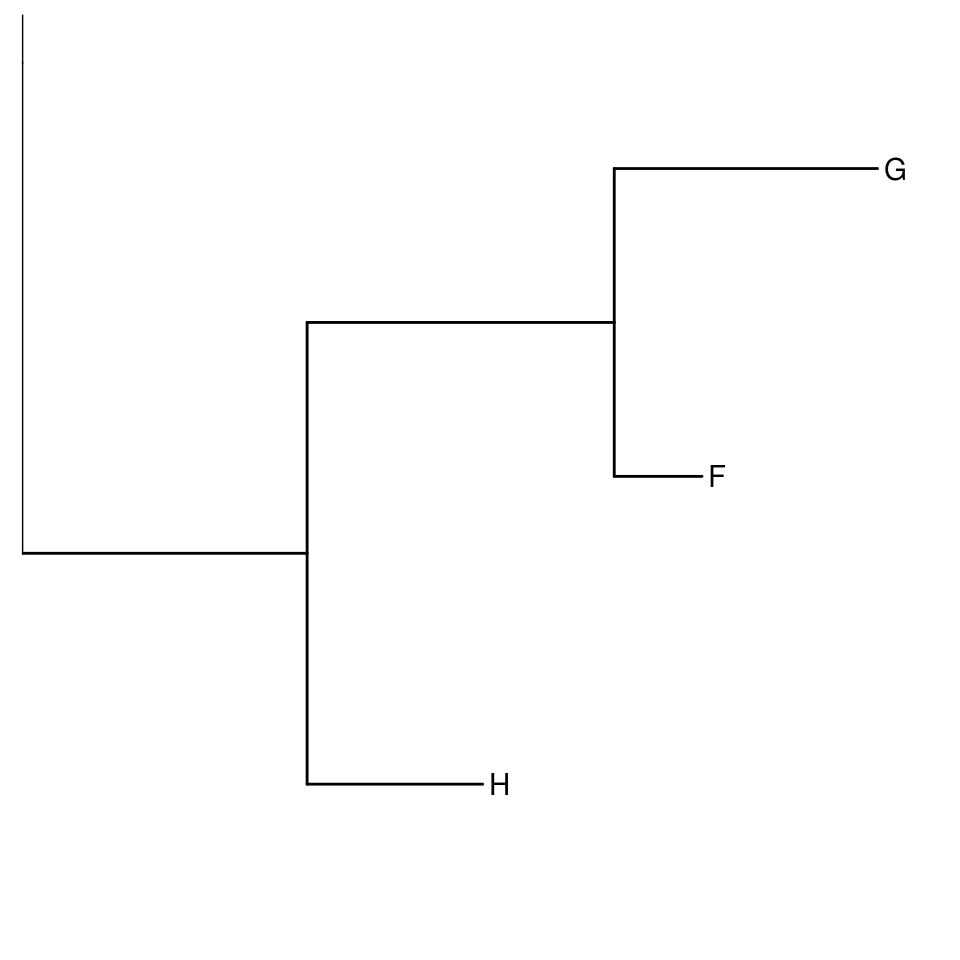The `ggtree` package defined several functions to manipulate tree view. `groupClade` and `groupOTU` methods were designed for clustering clades or related OTUs. `groupClade` accepts an internal node or a vector of internal nodes to cluster clade/clades.

Both `groupClade` and `groupOTU` work fine with tree and graphic object.

``````tree <- groupClade(tree, node=21)
ggtree(tree, aes(color=group, linetype=group))``````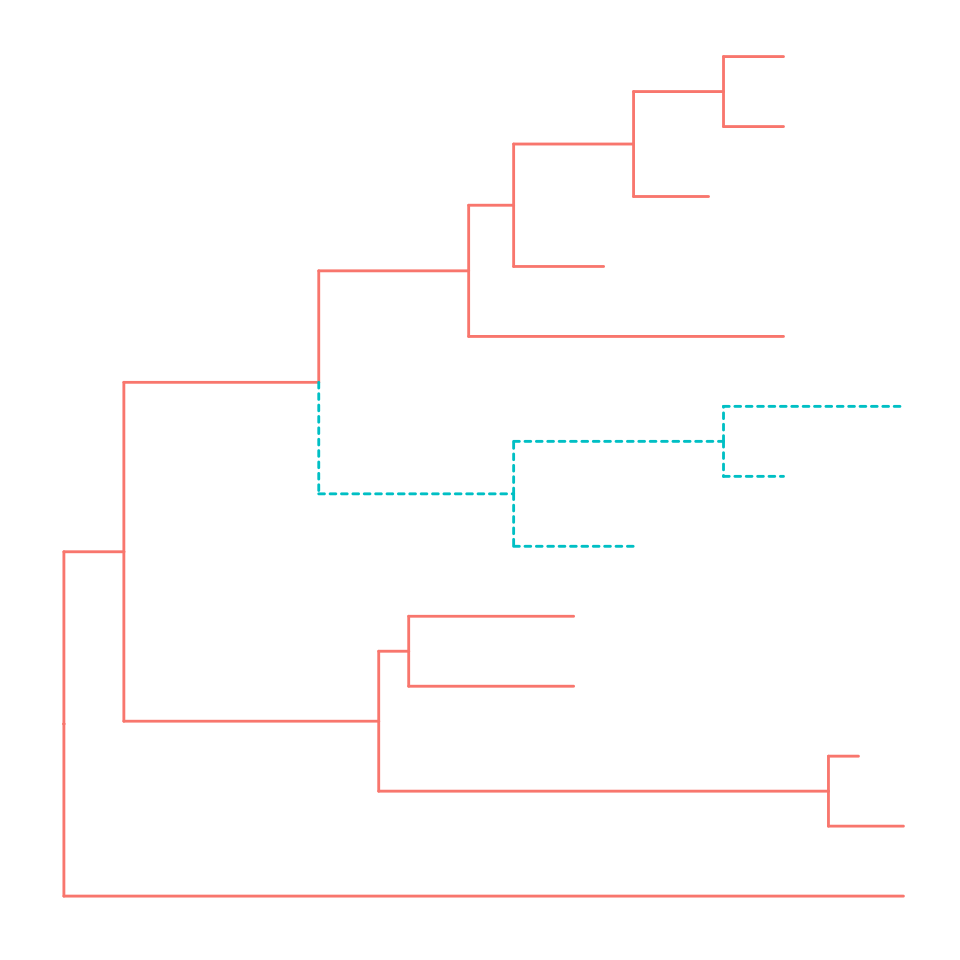The following command will produce the same figure.

``ggtree(read.tree(nwk)) %>% groupClade(node=21) + aes(color=group, linetype=group)``

With `groupClade` and `groupOTU`, it’s easy to highlight selected taxa and easy to select taxa to display related features.

``````tree <- groupClade(tree, node=c(21, 17))
ggtree(tree, aes(color=group, linetype=group)) + geom_tiplab(aes(subset=(group==2)))``````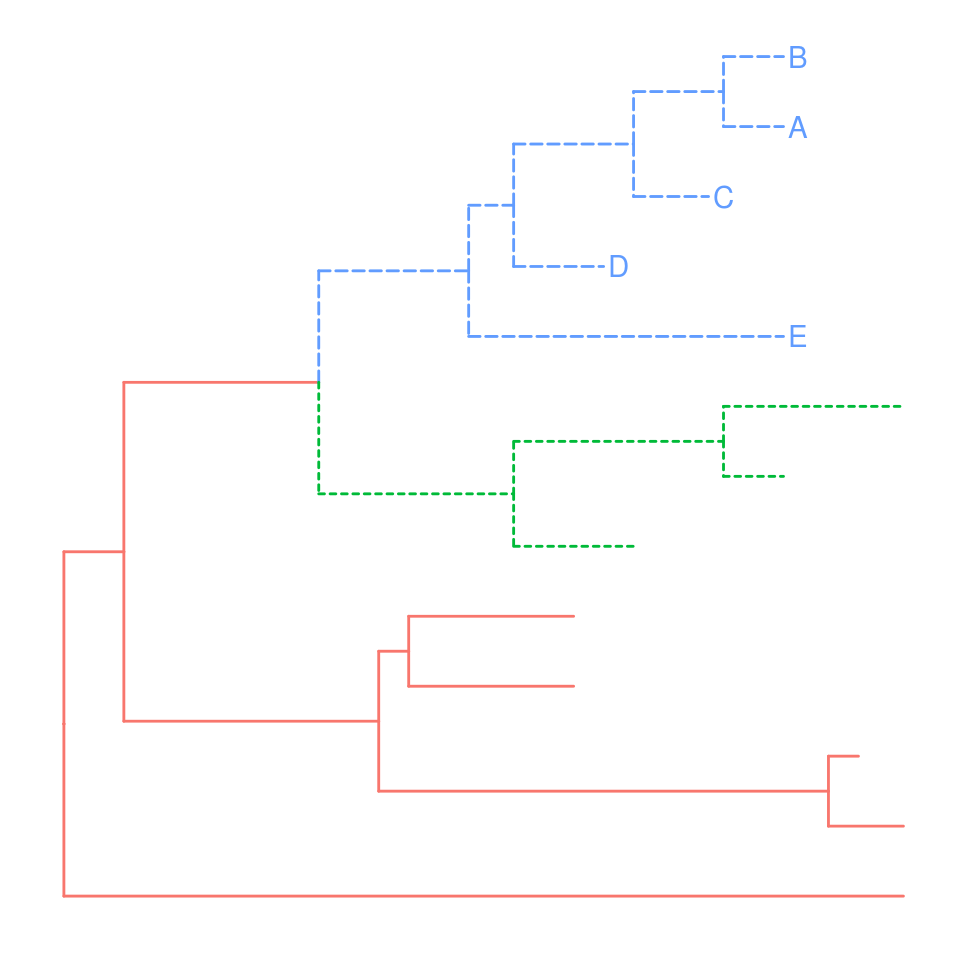# group OTUs

`groupOTU` accepts a vector of OTUs (taxa name) or a list of OTUs. `groupOTU` will trace back from OTUs to their most recent common ancestor and cluster them together. Related OTUs are not necessarily within a clade, they can be monophyletic (clade), polyphyletic or paraphyletic.

``tree <- groupOTU(tree, focus=c("D", "E", "F", "G"))``
``ggtree(tree, aes(color=group)) + geom_tiplab()``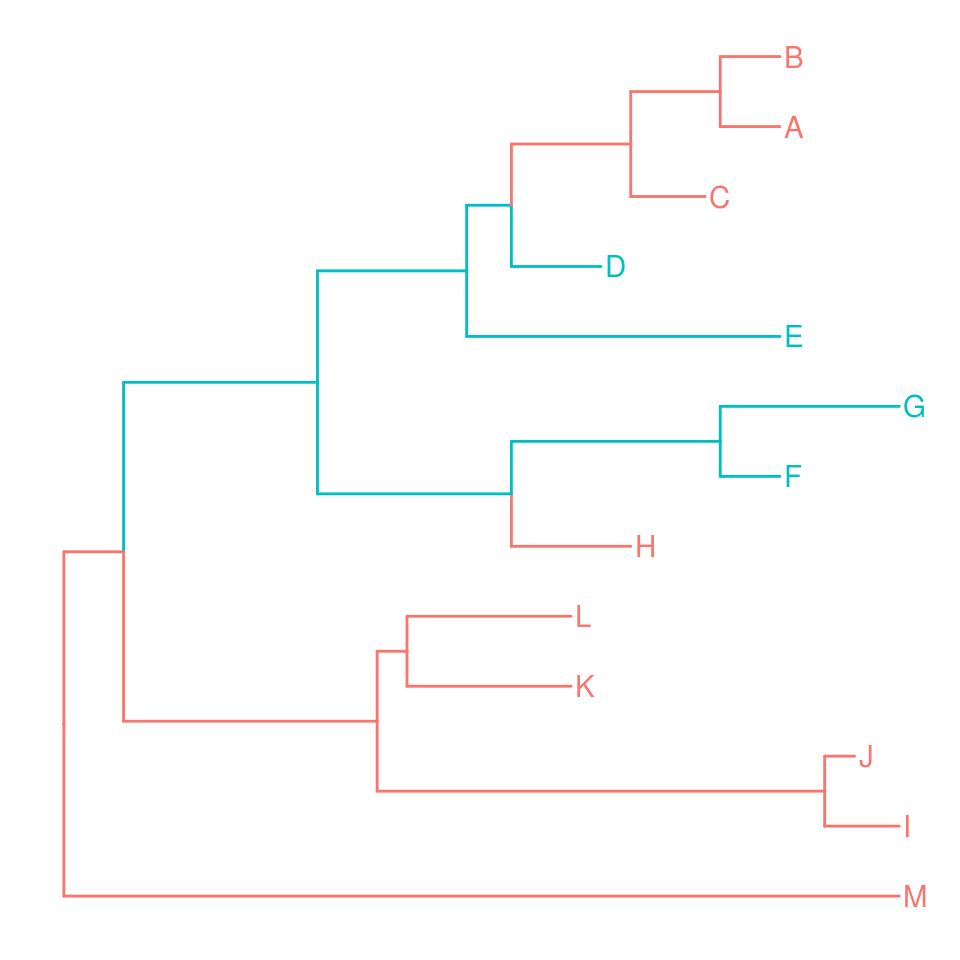`groupOTU` can also input a list of tip groups.

``````cls <- list(c1=c("A", "B", "C", "D", "E"),
c2=c("F", "G", "H"),
c3=c("L", "K", "I", "J"),
c4="M")

tree <- groupOTU(tree, cls)
library("colorspace")
ggtree(tree, aes(color=group, linetype=group)) + geom_tiplab() +
scale_color_manual(values=c("black", rainbow_hcl(4))) + theme(legend.position="right")``````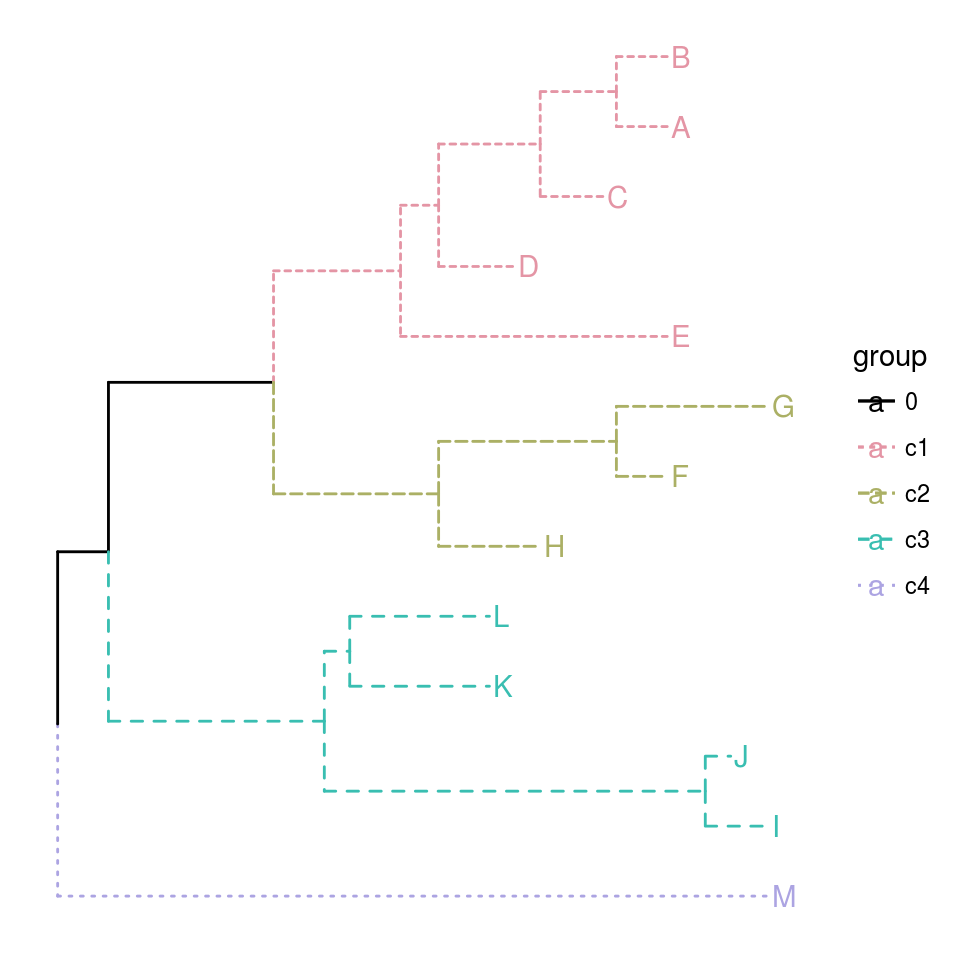`groupOTU` also work with graphic object.

``````p <- ggtree(tree)
groupOTU(p, LETTERS[1:5]) + aes(color=group) + geom_tiplab() + scale_color_manual(values=c("black", "firebrick"))``````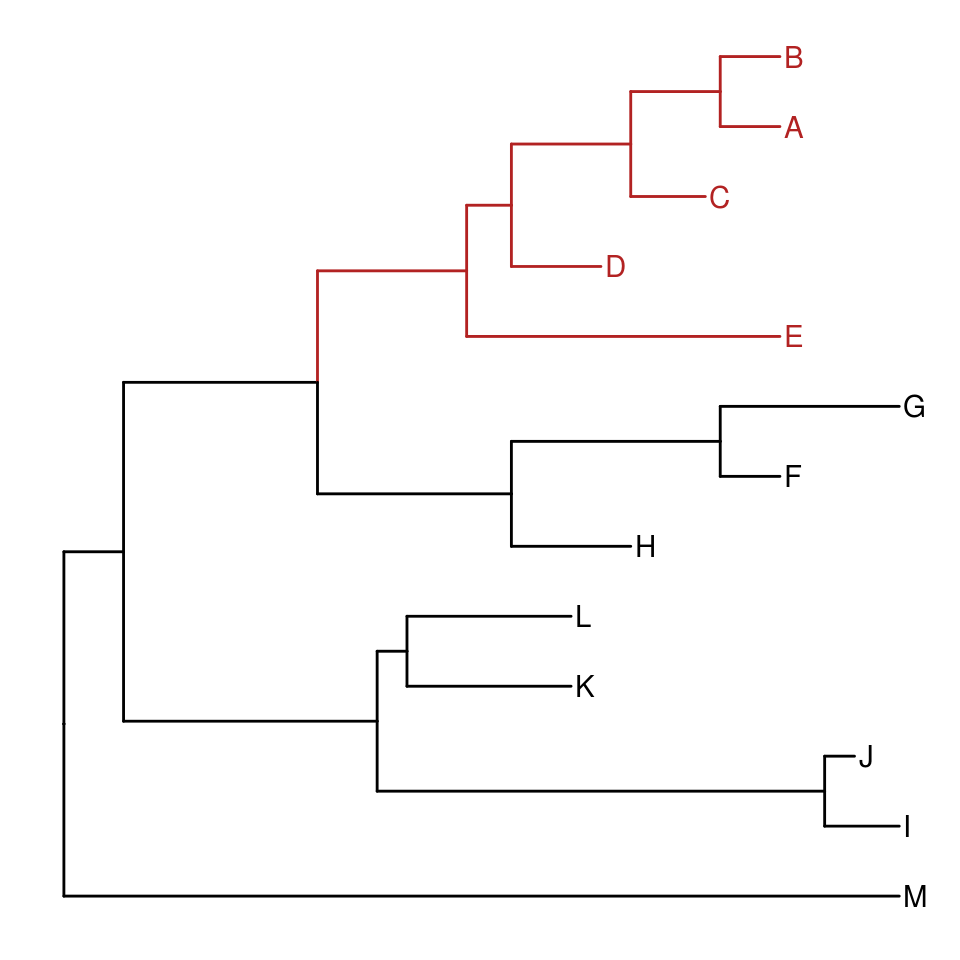The following example use `groupOTU` to display taxa classification.

``````library("ape")
data(chiroptera)
groupInfo <- split(chiroptera\$tip.label, gsub("_\\w+", "", chiroptera\$tip.label))
chiroptera <- groupOTU(chiroptera, groupInfo)
ggtree(chiroptera, aes(color=group), layout='circular') + geom_tiplab(size=1, aes(angle=angle))``````